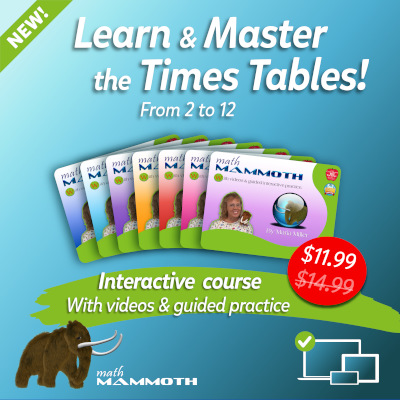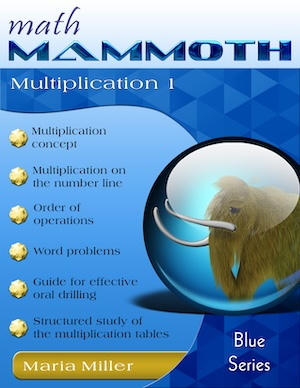GIVEAWAY! Win a Math Mammoth bundle or a grade level of your choice (13 winners!). Take part here. The giveaway runs through Sep 30, 2023.
^

# Multiplication tables from 2 to 12 — STRUCTURED drill — easy video lessonsLearn and Master the Times Tables! — a NEW INTERACTIVE course with videos & interactive exercises.

• Your child can master the tables once and for all!
• Teaching videos
• Structured drill
• Word problems
• Patterns & challenges
• Connections with concepts
→ Check it out!

You can use these videos and online activities to help students/children learn the multiplication tables from 2 to 12. They are presented in a specific order — the easiest ones first — which makes learning the tables MUCH easier.

 Table of 2  Interlude ↓ Table of 4  Interlude ↓ Table of 5  Interlude → Table of 3 Interlude ↓ Table of 6 Interlude ↓ Table of 11 Interlude → Table of 9 ↓ Table of 7 Interlude ↓ Table of 8 Interlude ↓ Table of 12 Ending Mathy's victory dance

Each teaching video has a similar structure:

1. First, we study the SKIP-COUNTING list or pattern for the specific table.
2. Next, I drill the table itself, but the STRUCTURE of the table is visible to the student during the drill. This ties the table with the just-learned skip-counting list, and also helps the student to use the CONCEPT of multiplication to possibly figure out some of the answers that are not yet memorized.
3. Lastly, we practice the table backwards. For example, I ask, "How many times 5 is 35?" This essentially teaches the basic division facts at the same time (though, I don't mention them as such).

This set of videos matches the lessons in my workbook Math Mammoth Multiplication 1, and Chapter 3 of Math Mammoth Grade 3 Complete Curriculum.

Also, practically all the lessons (table of 9 is an exception) are divided into two parts. The first part has to do with the drill of the particular multiplication table, and the second part contains varied multiplication practice, such as word problems, patterns, missing number exercises, and puzzles. I call those "second" parts "INTERLUDES" in the video list below.

You can read more about my approach to the memorization of times tables in my article on the topic.

As an additional practice tool, check out the online practice for multiplication tables and the interactive multiplication chart here at MathMammoth.com.### Math Mammoth Multiplication 1

A self-teaching worktext that covers the concept of multiplication from various angles, word problems, a guide for structural drilling, and a complete study of all 12 multiplication tables.

## Multiplication tables structured drill videos

### Table of 2

If you cannot see the video above, click here for an alternative video player.

Interlude: varied multiplication practice, word problems, doubles, even/odd

If you cannot see the video above, click here for an alternative video player.

### Table of 4

If you cannot see the video above, click here for an alternative video player.

Interlude: Comparing the multiplication tables of 2 and 4

If you cannot see the video above, click here for an alternative video player.

### Table of 5

If you cannot see the video above, click here for an alternative video player.

Interlude:

If you cannot see the video above, click here for an alternative video player.

### Table of 3

If you cannot see the video above, click here for an alternative video player.

Interlude: varied multiplication practice — missing factors, word problem, pattern

If you cannot see the video above, click here for an alternative video player.

### Table of 6

If you cannot see the video above, click here for an alternative video player.

Interlude: Doubling and halving tricks for the tables

If you cannot see the video above, click here for an alternative video player.

### Table of 11

If you cannot see the video above, click here for an alternative video player.

Interlude: missing factors and word problems

If you cannot see the video above, click here for an alternative video player.

### Table of 9

If you cannot see the video above, click here for an alternative video player.

### Table of 7

If you cannot see the video above, click here for an alternative video player.

Interlude: two multiplication patterns and a puzzle

If you cannot see the video above, click here for an alternative video player.

### Table of 8

If you cannot see the video above, click here for an alternative video player.

Interlude: missing factors, word problem, puzzle

If you cannot see the video above, click here for an alternative video player.

### Table of 12

If you cannot see the video above, click here for an alternative video player.

Ending: missing factors, multiplication pattern, and puzzles

If you cannot see the video above, click here for an alternative video player.

ENCORE: Multiplication tables VICTORY DANCE by Mathy, my mammoth mascot

If you cannot see the video above, click here for an alternative video player.### Math Mammoth Multiplication 1

A self-teaching worktext that covers the concept of multiplication from various angles, word problems, a guide for structural drilling, and a complete study of all 12 multiplication tables.

Math Mammoth Multiplication 1 — a self-teaching worktext with explanations & exercises for the concept of multiplication & all the tables

Math Mammoth Grade 3 complete curriculum

Learn 'Em Forwards and Backwards — a free online program by David Chandler that helps you practice and master the multiplication tables using the SAME order of learning the tables as in the above videos, in my book Math Mammoth Multiplication 1, and in Math Mammoth Grade 3 curriculum.

Back to all videos index

WAIT!

Receive my monthly collection of math tips & resources directly in your inbox — and get a FREE Math Mammoth book!You can unsubscribe at any time.

### Math Mammoth Tour

Confused about the different options? Take a virtual email tour around Math Mammoth! You'll receive:

An initial email to download your GIFT of over 400 free worksheets and sample pages from my books. Six other "TOURSTOP" emails that explain the important things and commonly asked questions concerning Math Mammoth curriculum. (Find out the differences between all these different-colored series!)

This way, you'll have time to digest the information over one or two weeks, plus an opportunity to ask me personally about the curriculum.
A monthly collection of math teaching tips & Math Mammoth updates (unsubscribe any time)### "Mini" Math Teaching Course

This is a little "virtual" 2-week course, where you will receive emails on important topics on teaching math, including:

- How to help a student who is behind
- Troubles with word problems
- Teaching multiplication tables
- Why fractions are so difficult
- The value of mistakes
- Should you use timed tests
- And more!

A GIFT of over 400 free worksheets and sample pages from my books right in the very beginning.A monthly collection of math teaching tips & Math Mammoth updates (unsubscribe any time)
Enter your email to receive math teaching tips, resources, Math Mammoth news & sales, humor, and more! I tend to send out these tips about once monthly, near the beginning of the month, but occasionally you may hear from me twice per month (and sometimes less often).• A GIFT of over 400 free worksheets and sample pages from my books.